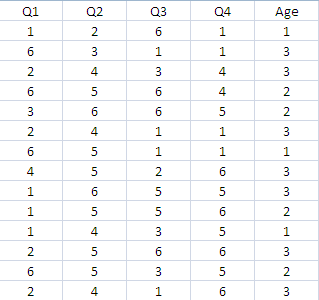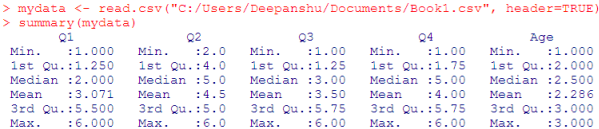# Data Exploration with R

This article demonstrates how to explore data with R. It is very important to explore data before starting to build a predictive model. It gives an idea about the structure of the dataset like number of continuous or categorical variables and number of observations (rows).

Dataset

The snapshot of the dataset used in this tutorial is pasted below. We have five variables - Q1, Q2, Q3, Q4 and Age. The variables Q1-Q4 represents survey responses of a questionnaire. The response lies between 1 and 6. The variable Age represents age groups of the respondents. It lies between 1 to 3. 1 represents Generation Z, 2 represents Generation X and Y, 3 represents Baby Boomers.Sample Data

Import data into R

The read.csv() function is used to import CSV file into R. The header = TRUE tells R that header is included in the data that we are going to import.
You can also create sample data which would be used further to demonstrate data exploration techniques. The program below creates random observations with replacement.
mydata = data.frame(Q1 = sample(1:6, 100, replace = TRUE),Q2 = sample(1:6, 100, replace = TRUE),Q3 = sample(1:6, 100, replace = TRUE), Q4 = sample(1:6, 100, replace = TRUE), Age = sample(1:3, 100, replace = TRUE))

1. Calculate basic descriptive statistics
summary(mydata)Data Exploration with R

To calculate summary of a particular column, say third column, you can use the following syntax :
summary( mydata)
To calculate summary of a particular column by its name, you can use the following syntax :
summary( mydata\$Q1)

2. Lists name of variables in a dataset
names(mydata)
```> names(mydata)
 "Q1"  "Q2"  "Q3"  "Q4"  "Age"
```

3. Calculate number of rows in a dataset
nrow(mydata)
```> nrow(mydata)
 100
```
4. Calculate number of columns in a dataset
ncol(mydata)
```> ncol(mydata)
 5
```
5. List structure of a dataset
str(mydata)
```> str(mydata)
'data.frame': 100 obs. of  5 variables:
\$ Q1 : int  1 5 3 1 6 2 2 1 4 1 ...
\$ Q2 : int  3 3 3 1 1 4 2 2 6 1 ...
\$ Q3 : int  4 2 1 4 3 6 1 4 4 4 ...
\$ Q4 : int  3 5 1 1 3 4 2 2 5 1 ...
\$ Age: int  3 1 1 1 1 1 3 1 2 3 ...
```
6. See first 6 rows of dataset
```  Q1 Q2 Q3 Q4 Age
1  1  3  4  3   3
2  5  3  2  5   1
3  3  3  1  1   1
4  1  1  4  1   1
5  6  1  3  3   1
6  2  4  6  4   1
```

7. First n rows of dataset

In the code below, we are selecting first 5 rows of dataset.
8. All rows but the last row
9. Last 6 rows of dataset
tail(mydata)
10. Last n rows of dataset

In the code below, we are selecting last 5 rows of dataset.
tail(mydata, n=5)
11. All rows but the first row
tail(mydata, n= -1)

12. Select random rows from a dataset
library(dplyr)
sample_n(mydata, 5)
If dplyr package is not already installed, make sure you install it before running the above script.

13. Selecting N% random rows
library(dplyr)
sample_frac(mydata, 0.1)
In this case, it selects 10% random rows from mydata data frame.

14. Number of missing values

The function below returns number of missing values in each variable of a dataset.
colSums(is.na(mydata))
It can also be written like -
sapply(mydata, function(y) sum(is.na(y)))

15. Number of missing values in a single variable
sum(is.na(mydata\$Q1))
Related Posts
ShareDeepanshu founded ListenData with a simple objective - Make analytics easy to understand and follow. He has over 10 years of experience in data science. During his tenure, he worked with global clients in various domains like Banking, Insurance, Private Equity, Telecom and HR.

3 Responses to "Data Exploration with R"
1.#to create the data used in this tutorial, use following command
mydata = data.frame(Q1 = sample(1:6, 15, replace = TRUE),Q2 = sample(1:6, 15, replace = TRUE),Q3 = sample(1:6, 15, replace = TRUE), Q4 = sample(1:6, 15, replace = TRUE), Age = sample(1:3, 15, replace = TRUE))

1.Thanks for pointing it out. I have added it to this post. Cheers!

2.Great article!

Next → ← Prev